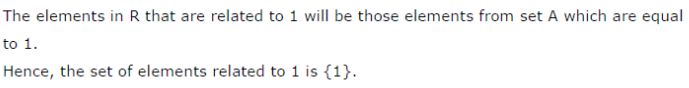# Show that each of the relation R in the set A = { x ∈Z: 0≤x≤12}, A={x} given by

Show that each of the relation R in the set A = { x ∈Z: 0≤x≤12}, A={x} given by
(i) R = { (a,b) : |a - b| is a multiple of 4}
(ii) R = {(a,b):a = b} is an equivalence relation.
Find the set of all elements related to 1 in each case.